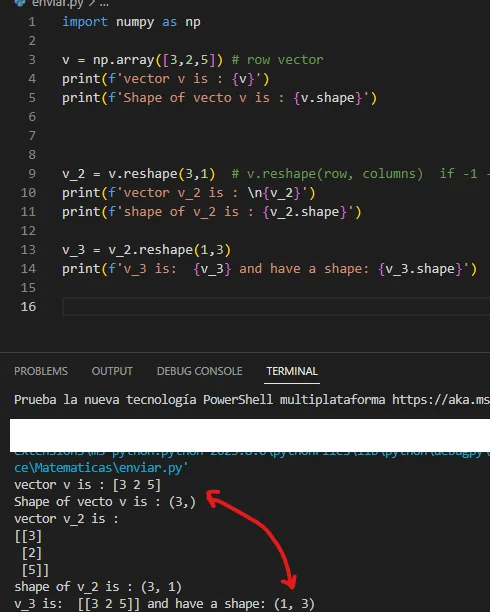Posted on:

24 May 2023

1

Good afternoon.
During the video it is commented that when creating a vector, by default this is a row vector.
For example-->v = np.array([3,2,5])

This vector will have the form -->[3,2,5]  ---> ( 1 row ,3 colums)
And when we use the print(v) function, it has that output.
However using v.shape , we get (3,)

Why we get (3,) instead of ( 1 ,3)--> if 1st element refers rows ans second to the colums.

And why before do v_3= v_2 .reshape(1.3) to v_2 --->  v_3 is equal v, why ¿v_3.shape --> (1,3)

Does this(3,) correspond to 3 rows?